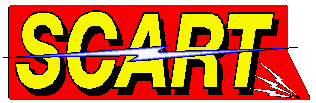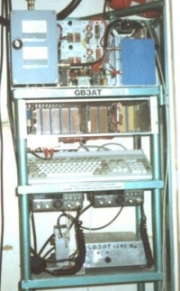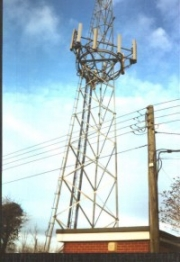GB3AT - SU 451293

 GB3AT is located just west of Winchester Hampshire. Its grid reference is SU451293 with aerials at 6 meters above ground level. There are 4 plate aerials facing SW, SE, NW and NE, all 4 are on for TX. On RX the aerials are switched one by one, with the one with the strongest signal being selected and switched on. The input is 1249Mhz with the output on 1316Mhz. The software was written by Ian G6HNJ for a Amiga 1200 which also controls all the hardware. It is hopped to developed this repeater to include teletext stile pages and a message board for user to leave a message for other users. There are also some more hardware projects to be finished, to include a sinc reprocessor and "P" grade meter.GB3AT in its rack GB3ATs mast before the aerials were installed

Quick Look Up Chart For GB3AT

To log onto GB3AT you need to use 433.750Mhz and DTMF. The commands are as follows :-

 # Login 0=48 1=49 2=50 3=51 4=52 5=53 6=54 * Enter a Command 7=55 8=56 9=57 0 Show Usage Chart 1 Show Call Log A=65 B=66 C=67 D=68 E=69 F=70 G=71 4 Goto Previous Page H=72 I=73 J=74 K=75 L=76 M=77 N=78 6 Goto Next Page O=79 P=80 Q=81 R=82 S=83 T=84 U=85 99 End V=86 W=87 X=88 Y=89 Z=90

So to logon you would type #yourcall99 i.e. for G7JTT it would be # 71 55 74 84 84 99

 GB3AT coverage map(P3 and above should be expected with in the colour shading)

 News

Back